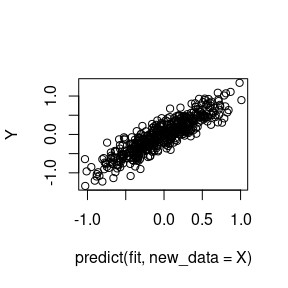# Fitting the Highly Adaptive Lasso with hal9001

## Introduction

The highly adaptive Lasso (HAL) is a flexible machine learning algorithm that nonparametrically estimates a function based on available data by embedding a set of input observations and covariates in an extremely high-dimensional space (i.e., generating basis functions from the available data). For an input data matrix of $$n$$ observations and $$d$$ covariates, the maximum number of zero-order basis functions generated is approximately $$n \cdot 2^{d - 1}$$. To select a set of basis functions from among the (possibly reduced/screener) set that’s generated, the lasso is employed. The hal9001 R package (Hejazi, Coyle, and van der Laan 2020; Coyle, Hejazi, and van der Laan, n.d.) provides an efficient implementation of this routine, relying on the glmnet R package (Friedman, Hastie, and Tibshirani 2010) for compatibility with the canonical Lasso implementation and using lasso regression with an input matrix composed of basis functions. Consult Benkeser and van der Laan (2016), (vdl2015generally?), van der Laan (2017) for detailed theoretical descriptions of HAL and its various optimality properties.

## Preliminaries

library(data.table)
library(ggplot2)
# simulation constants
set.seed(467392)
n_obs <- 500
n_covars <- 3

# make some training data
x <- replicate(n_covars, rnorm(n_obs))
y <- sin(x[, 1]) + sin(x[, 2]) + rnorm(n_obs, mean = 0, sd = 0.2)

# make some testing data
test_x <- replicate(n_covars, rnorm(n_obs))
test_y <- sin(x[, 1]) + sin(x[, 2]) + rnorm(n_obs, mean = 0, sd = 0.2)

Let’s look at simulated data:

head(x)
##             [,1]       [,2]       [,3]
## [1,]  2.44102981 -0.4337909  0.4670282
## [2,] -1.21932335  0.3336395  0.8894277
## [3,] -0.40613567 -0.3869374  0.3474353
## [4,] -1.09760477 -1.4663219 -0.1173214
## [5,]  0.23710498  1.2565812  1.8049389
## [6,]  0.06810091 -0.7020905  0.9301941
head(y)
##   0.2372289 -0.6023415 -0.7569124 -1.8021339  1.0589707 -0.3373555

## Using the Highly Adaptive Lasso

library(hal9001)
## Loading required package: Rcpp
## hal9001 v0.4.6: The Scalable Highly Adaptive Lasso
## note: fit_hal defaults have changed. See ?fit_hal for details

### Fitting the model

HAL uses the popular glmnet R package for the lasso step:

hal_fit <- fit_hal(X = x, Y = y)

### Obtaining model predictions

# training sample prediction for HAL vs HAL9000
mse <- function(preds, y) {
mean((preds - y)^2)
}

preds_hal <- predict(object = hal_fit, new_data = x)
mse_hal <- mse(preds = preds_hal, y = y)
mse_hal
##  0.04499671
oob_hal <- predict(object = hal_fit, new_data = test_x)
oob_hal_mse <- mse(preds = oob_hal, y = test_y)
oob_hal_mse
##  1.801073

### Reducing basis functions

As described in Benkeser and van der Laan (2016), the HAL algorithm operates by first constructing a set of basis functions and subsequently fitting a Lasso model with this set of basis functions as the design matrix. Several approaches are considered for reducing this set of basis functions: 1. Removing duplicated basis functions (done by default in the fit_hal function), 2. Removing basis functions that correspond to only a small set of observations; a good rule of thumb is to scale with $$\frac{1}{\sqrt{n}}$$, and that is the default.

The second of these two options may be modified by specifying the reduce_basis argument to the fit_hal function:

hal_fit_reduced <- fit_hal(X = x, Y = y, reduce_basis = 0.1)
## Warning in fit_hal(X = x, Y = y, reduce_basis = 0.1): Dropping reduce_basis;
## only applies if smoothness_orders = 0
hal_fit_reduced$times ## user.self sys.self elapsed user.child sys.child ## enumerate_basis 0.004 0.000 0.004 0 0 ## design_matrix 0.014 0.000 0.015 0 0 ## reduce_basis 0.001 0.000 0.000 0 0 ## remove_duplicates 0.000 0.000 0.000 0 0 ## lasso 1.568 0.008 1.576 0 0 ## total 1.587 0.008 1.595 0 0 In the above, all basis functions with fewer than 10% of observations meeting the criterion imposed are automatically removed prior to the Lasso step of fitting the HAL regression. The results appear below summary(hal_fit_reduced)$table
##              coef
##  1: -1.346994e+00
##  2:  2.576756e-01
##  3: -2.434120e-01
##  4:  2.336262e-01
##  5:  1.706263e-01
##  6:  1.590289e-01
##  7: -1.461021e-01
##  8: -1.123958e-01
##  9: -9.993951e-02
## 10: -9.592210e-02
## 11:  7.420728e-02
## 12:  6.807883e-02
## 13: -4.751534e-02
## 14: -4.445773e-02
## 15:  4.390066e-02
## 16: -4.258466e-02
## 17:  4.088055e-02
## 18: -3.946385e-02
## 19:  3.810985e-02
## 20: -2.172250e-02
## 21:  1.750069e-02
## 22: -1.562506e-02
## 23:  1.279327e-02
## 24: -1.260048e-02
## 25: -1.229455e-02
## 26: -1.056098e-02
## 27: -6.209854e-03
## 28:  5.314720e-03
## 29:  3.614975e-03
## 30:  1.318925e-03
## 31: -9.520939e-04
## 32:  7.387511e-04
## 33: -4.744200e-05
## 34:  9.489334e-06
## 35:  6.773258e-06
##              coef
##                                                                                                                term
##  1:                                                                                                     (Intercept)
##  2:                                                                             [ I(x2 >= -1.332)*(x2 - -1.332)^1 ]
##  3:                                         [ I(x2 >= 1.603)*(x2 - 1.603)^1 ] * [ I(x3 >= -3.289)*(x3 - -3.289)^1 ]
##  4:                                       [ I(x1 >= -0.962)*(x1 - -0.962)^1 ] * [ I(x3 >= -3.289)*(x3 - -3.289)^1 ]
##  5:                                       [ I(x2 >= -1.583)*(x2 - -1.583)^1 ] * [ I(x3 >= -3.289)*(x3 - -3.289)^1 ]
##  6:                                       [ I(x1 >= -1.425)*(x1 - -1.425)^1 ] * [ I(x2 >= -3.038)*(x2 - -3.038)^1 ]
##  7:                                         [ I(x1 >= 1.629)*(x1 - 1.629)^1 ] * [ I(x2 >= -3.038)*(x2 - -3.038)^1 ]
##  8:                                         [ I(x1 >= 1.368)*(x1 - 1.368)^1 ] * [ I(x3 >= -3.289)*(x3 - -3.289)^1 ]
##  9:                                         [ I(x1 >= 0.943)*(x1 - 0.943)^1 ] * [ I(x3 >= -3.289)*(x3 - -3.289)^1 ]
## 10:                                         [ I(x2 >= 1.017)*(x2 - 1.017)^1 ] * [ I(x3 >= -3.289)*(x3 - -3.289)^1 ]
## 11:                                                                             [ I(x2 >= -1.592)*(x2 - -1.592)^1 ]
## 12:                                       [ I(x2 >= -0.702)*(x2 - -0.702)^1 ] * [ I(x3 >= -3.289)*(x3 - -3.289)^1 ]
## 13:                                         [ I(x2 >= 0.695)*(x2 - 0.695)^1 ] * [ I(x3 >= -3.289)*(x3 - -3.289)^1 ]
## 14:                                         [ I(x1 >= 1.135)*(x1 - 1.135)^1 ] * [ I(x2 >= -3.038)*(x2 - -3.038)^1 ]
## 15:   [ I(x1 >= -3.224)*(x1 - -3.224)^1 ] * [ I(x2 >= 1.162)*(x2 - 1.162)^1 ] * [ I(x3 >= -0.497)*(x3 - -0.497)^1 ]
## 16:                                       [ I(x2 >= -3.038)*(x2 - -3.038)^1 ] * [ I(x3 >= -3.289)*(x3 - -3.289)^1 ]
## 17:                                                                             [ I(x1 >= -1.866)*(x1 - -1.866)^1 ]
## 18: [ I(x1 >= -3.224)*(x1 - -3.224)^1 ] * [ I(x2 >= -3.038)*(x2 - -3.038)^1 ] * [ I(x3 >= -3.289)*(x3 - -3.289)^1 ]
## 19:                                       [ I(x1 >= -3.224)*(x1 - -3.224)^1 ] * [ I(x2 >= -0.976)*(x2 - -0.976)^1 ]
## 20:                                                                             [ I(x3 >= -3.289)*(x3 - -3.289)^1 ]
## 21:     [ I(x1 >= -0.313)*(x1 - -0.313)^1 ] * [ I(x2 >= 0.12)*(x2 - 0.12)^1 ] * [ I(x3 >= -3.289)*(x3 - -3.289)^1 ]
## 22:   [ I(x1 >= 0.307)*(x1 - 0.307)^1 ] * [ I(x2 >= -3.038)*(x2 - -3.038)^1 ] * [ I(x3 >= -3.289)*(x3 - -3.289)^1 ]
## 23:   [ I(x1 >= -3.224)*(x1 - -3.224)^1 ] * [ I(x2 >= 0.495)*(x2 - 0.495)^1 ] * [ I(x3 >= -0.256)*(x3 - -0.256)^1 ]
## 24: [ I(x1 >= -0.901)*(x1 - -0.901)^1 ] * [ I(x2 >= -3.038)*(x2 - -3.038)^1 ] * [ I(x3 >= -0.048)*(x3 - -0.048)^1 ]
## 25:                                         [ I(x1 >= -3.224)*(x1 - -3.224)^1 ] * [ I(x2 >= 1.603)*(x2 - 1.603)^1 ]
## 26:   [ I(x1 >= -3.224)*(x1 - -3.224)^1 ] * [ I(x2 >= 0.495)*(x2 - 0.495)^1 ] * [ I(x3 >= -3.289)*(x3 - -3.289)^1 ]
## 27: [ I(x1 >= -0.901)*(x1 - -0.901)^1 ] * [ I(x2 >= -0.374)*(x2 - -0.374)^1 ] * [ I(x3 >= -3.289)*(x3 - -3.289)^1 ]
## 28:   [ I(x1 >= -3.224)*(x1 - -3.224)^1 ] * [ I(x2 >= -3.038)*(x2 - -3.038)^1 ] * [ I(x3 >= 0.596)*(x3 - 0.596)^1 ]
## 29:                                       [ I(x1 >= -0.687)*(x1 - -0.687)^1 ] * [ I(x3 >= -3.289)*(x3 - -3.289)^1 ]
## 30:   [ I(x1 >= -3.224)*(x1 - -3.224)^1 ] * [ I(x2 >= -3.038)*(x2 - -3.038)^1 ] * [ I(x3 >= 0.374)*(x3 - 0.374)^1 ]
## 31:                                         [ I(x1 >= 0.738)*(x1 - 0.738)^1 ] * [ I(x3 >= -3.289)*(x3 - -3.289)^1 ]
## 32:                                                                             [ I(x2 >= -1.412)*(x2 - -1.412)^1 ]
## 33: [ I(x1 >= -0.901)*(x1 - -0.901)^1 ] * [ I(x2 >= -3.038)*(x2 - -3.038)^1 ] * [ I(x3 >= -3.289)*(x3 - -3.289)^1 ]
## 34:                                                                             [ I(x2 >= -1.235)*(x2 - -1.235)^1 ]
## 35: [ I(x1 >= -3.224)*(x1 - -3.224)^1 ] * [ I(x2 >= -0.374)*(x2 - -0.374)^1 ] * [ I(x3 >= -3.289)*(x3 - -3.289)^1 ]
##                                                                                                                term

Other approaches exist for reducing the set of basis functions before they are actually created, which is essential for most real-world applications with HAL. Currently, we provide this “pre-screening” via num_knots argument in hal_fit. The num_knots argument is akin to binning: it increases the coarseness of the approximation. num_knots allows one to specify the number of knot points used to generate the basis functions for each/all interaction degree(s). This reduces the total number of basis functions generated, and thus the size of the optimization problem, and it can dramatically decrease runtime. One can pass in a vector of length max_degree to num_knots, specifying the number of knot points to use by interaction degree for each basis function. Thus, one can specify if interactions of higher degrees (e.g., two- or three- way interactions) should be more coarse. Increasing the coarseness of more complex basis functions helps prevent a combinatorial explosion of basis functions, which can easily occur when basis functions are generated for all possible knot points. We will show an example with num_knots in the section that follows.

### Specifying smoothness of the HAL model

One might wish to enforce smoothness on the functional form of the HAL fit. This can be done using the smoothness_orders argument. Setting smoothness_orders = 0 gives a piece-wise constant fit (via zero-order basis functions), allowing for discontinuous jumps in the function. This is useful if one does not want to assume any smoothness or continuity of the “true” function. Setting smoothness_orders = 1 gives a piece-wise linear fit (via first-order basis functions), which is continuous and mostly differentiable. In general, smoothness_orders = k corresponds to a piece-wise polynomial fit of degree $$k$$. Mathematically, smoothness_orders = k corresponds with finding the best fit under the constraint that the total variation of the function’s $$k^{\text{th}}$$ derivative is bounded by some constant, which is selected with cross-validation.

Let’s see this in action.

set.seed(98109)
num_knots <- 100 # Try changing this value to see what happens.
n_covars <- 1
n_obs <- 250
x <- replicate(n_covars, runif(n_obs, min = -4, max = 4))
y <- sin(x[, 1]) + rnorm(n_obs, mean = 0, sd = 0.2)
ytrue <- sin(x[, 1])

hal_fit_0 <- fit_hal(
X = x, Y = y, smoothness_orders = 0, num_knots = num_knots
)

hal_fit_smooth_1 <- fit_hal(
X = x, Y = y, smoothness_orders = 1, num_knots = num_knots
)

hal_fit_smooth_2_all <- fit_hal(
X = x, Y = y, smoothness_orders = 2, num_knots = num_knots,
fit_control = list(cv_select = FALSE)
)

hal_fit_smooth_2 <- fit_hal(
X = x, Y = y, smoothness_orders = 2, num_knots = num_knots
)

pred_0 <- predict(hal_fit_0, new_data = x)
pred_smooth_1 <- predict(hal_fit_smooth_1, new_data = x)
pred_smooth_2 <- predict(hal_fit_smooth_2, new_data = x)

pred_smooth_2_all <- predict(hal_fit_smooth_2_all, new_data = x)
dt <- data.table(x = as.vector(x))
dt <- cbind(dt, pred_smooth_2_all)
long <- melt(dt, id = "x")
ggplot(long, aes(x = x, y = value, group = variable)) +
geom_line()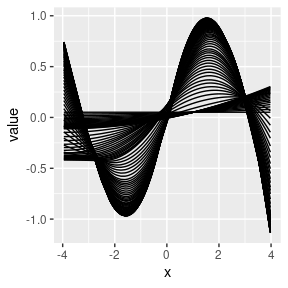Comparing the mean squared error (MSE) between the predictions and the true (denoised) outcome, the first- and second- order smoothed HAL is able to recover from the coarseness of the basis functions caused by the small num_knots argument. Also, the HAL with second-order smoothness is able to fit the true function very well (as expected, since sin(x) is a very smooth function). The main benefit of imposing higher-order smoothness is that fewer knot points are required for a near-optimal fit. Therefore, one can safely pass a smaller value to num_knots for a big decrease in runtime without sacrificing performance.

mean((pred_0 - ytrue)^2)
##  0.007635298
mean((pred_smooth_1 - ytrue)^2)
##  0.002864562
mean((pred_smooth_2 - ytrue)^2)
##  0.001804221
dt <- data.table(
x = as.vector(x),
ytrue = ytrue,
y = y,
pred0 = pred_0,
pred1 = pred_smooth_1,
pred2 = pred_smooth_2
)
long <- melt(dt, id = "x")
ggplot(long, aes(x = x, y = value, color = variable)) +
geom_line()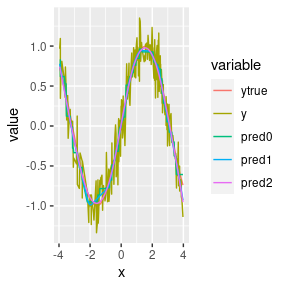plot(x, pred_0, main = "Zero order smoothness fit")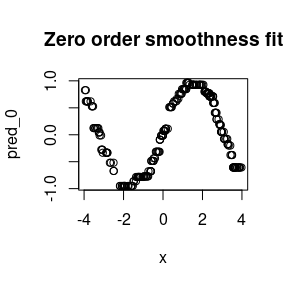plot(x, pred_smooth_1, main = "First order smoothness fit")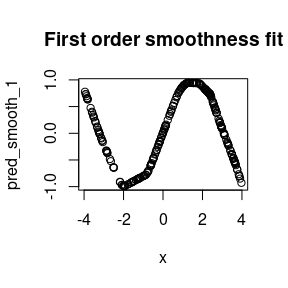plot(x, pred_smooth_2, main = "Second order smoothness fit")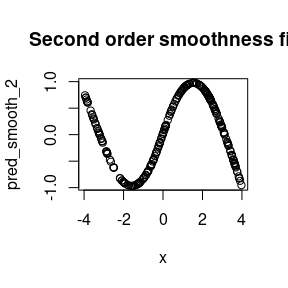In general, if the basis functions are not coarse, then the performance for different smoothness orders is similar. Notice how the runtime is a fair bit slower when more knot points are considered. In general, we recommend either zero- or first- order smoothness. Second-order smoothness tends to be less robust and suffers from extrapolation on new data. One can also use cross-validation to data-adaptively choose the optimal smoothness (invoked in fit_hal by setting adaptive_smoothing = TRUE). Comparing the following simulation and the previous one, the HAL with second-order smoothness performed better when there were fewer knot points.

set.seed(98109)
n_covars <- 1
n_obs <- 250
x <- replicate(n_covars, runif(n_obs, min = -4, max = 4))
y <- sin(x[, 1]) + rnorm(n_obs, mean = 0, sd = 0.2)
ytrue <- sin(x[, 1])

hal_fit_0 <- fit_hal(
X = x, Y = y, smoothness_orders = 0, num_knots = 100
)
hal_fit_smooth_1 <- fit_hal(
X = x, Y = y, smoothness_orders = 1, num_knots = 100
)

hal_fit_smooth_2 <- fit_hal(
X = x, Y = y, smoothness_orders = 2, num_knots = 100
)

pred_0 <- predict(hal_fit_0, new_data = x)
pred_smooth_1 <- predict(hal_fit_smooth_1, new_data = x)
pred_smooth_2 <- predict(hal_fit_smooth_2, new_data = x)
mean((pred_0 - ytrue)^2)
##  0.007635298
mean((pred_smooth_1 - ytrue)^2)
##  0.002864562
mean((pred_smooth_2 - ytrue)^2)
##  0.001859842
plot(x, pred_0, main = "Zero order smoothness fit")plot(x, pred_smooth_1, main = "First order smoothness fit")plot(x, pred_smooth_2, main = "Second order smoothness fit")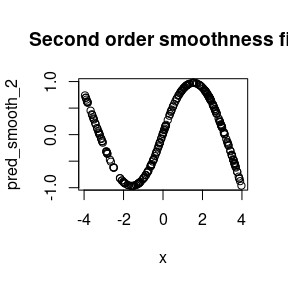### Formula interface

One might wish to specify the functional form of the HAL fit further. This can be done using the formula interface. Specifically, the formula interface allows one to specify monotonicity constraints on components of the HAL fit. It also allows one to specify exactly which basis functions (e.g., interactions) one wishes to model. The formula_hal function generates a formula object from a user-supplied character string, and this formula object contains the necessary specification information for fit_hal and glmnet. The formula_hal function is intended for use within fit_hal, and the user-supplied character string is inputted into fit_hal. Here, we call formula_hal directly for illustrative purposes.

set.seed(98109)
num_knots <- 100

n_obs <- 500
x1 <- runif(n_obs, min = -4, max = 4)
x2 <- runif(n_obs, min = -4, max = 4)
A <- runif(n_obs, min = -4, max = 4)
X <- data.frame(x1 = x1, x2 = x2, A = A)
Y <- rowMeans(sin(X)) + rnorm(n_obs, mean = 0, sd = 0.2)

We can specify an additive model in a number of ways.

The formula below includes the outcome, but formula_hal doesn’t fit a HAL model, and doesn’t need the outcome (actually everything before “$$\tilde$$” is ignored in formula_hal). This is why formula_hal takes the input X matrix of covariates, and not X and Y. In what follows, we include formulas with and without “y” in the character string.

# The h function is used to specify the basis functions for a given term
# h(x1) generates one-way basis functions for the variable x1
# This is an additive model:
formula <- ~ h(x1) + h(x2) + h(A)
# We can actually evaluate the h function as well. We need to specify some tuning parameters in the current environment:
smoothness_orders <- 0
num_knots <- 10
# It will look in the parent environment for X and the above tuning parameters
form_term <- h(x1) + h(x2) + h(A)
form_term$basis_list[] ##$cols
##  1
##
## $cutoffs ##  -3.971502 ## ##$orders
##  0
# We don't need the variables in the parent environment if we specify them directly:
rm(smoothness_orders)
rm(num_knots)
# h excepts the arguments s and k. s stands for smoothness and is equivalent to smoothness_orders in use. k specifies the number of knots. 
form_term_new <- h(x1, s = 0, k = 10) + h(x2, s = 0, k = 10) + h(A, s = 0, k = 10)
# They are the same!
length(form_term_new$basis_list) == length(form_term$basis_list)
##  TRUE
# To evaluate a unevaluated formula object like:
formula <- ~ h(x1) + h(x2) + h(A)
# we can use the formula_hal function:
formula <- formula_hal(
~ h(x1) + h(x2) + h(A),
X = X, smoothness_orders = 1, num_knots = 10
)
# Note that the arguments smoothness_orders and/or num_knots will not be used if s and/or k are specified in h.
formula <- formula_hal(
Y ~ h(x1, k = 1) + h(x2, k = 1) + h(A, k = 1),
X = X, smoothness_orders = 1, num_knots = 10
)

The . argument. We can generate an additive model for all or a subset of variables using the . variable and . argument of h. By default, . in h(.) is treated as a wildcard and basis functions are generated by replacing the . with all variables in X.

smoothness_orders <- 1
num_knots <- 5
colnames(X)
##  "x1" "x2" "A"
# Shortcut:
formula1 <- h(.)
# Longcut:
formula2 <- h(x1) + h(x2) + h(A)
# Same number of basis functions
length(formula1$basis_list) == length(formula2$basis_list)
##  TRUE
# Maybe we only want an additive model for x1 and x2
# Use the . argument
formula1 <- h(., . = c("x1", "x2"))
formula2 <- h(x1) + h(x2)
length(formula1$basis_list) == length(formula2$basis_list)
##  TRUE

We can specify interactions as follows.

#  Two way interactions
formula1 <- h(x1) + h(x2) + h(A) + h(x1, x2)
formula2 <- h(.) + h(x1, x2)
length(formula1$basis_list) == length(formula2$basis_list)
##  TRUE
#
formula1 <- h(.) + h(x1, x2) + h(x1, A) + h(x2, A)
formula2 <- h(.) + h(., .)
length(formula1$basis_list) == length(formula2$basis_list)
##  TRUE
#  Three way interactions
formula1 <- h(.) + h(., .) + h(x1, A, x2)
formula2 <- h(.) + h(., .) + h(., ., .)
length(formula1$basis_list) == length(formula2$basis_list)
##  TRUE

Sometimes, one might want to build an additive model, but include all two-way interactions with one variable (e.g., treatment “A”). This can be done in a variety of ways. The . argument allows you to specify a subset of variables.

# Write it all out
formula <- h(x1) + h(x2) + h(A) + h(A, x1) + h(A, x2)

# interactions
formula <- y ~ h(.) + h(A, x1) + h(A, x2)

# Use the "wildcard" feature for when "." is included in the "h()" term. This
# useful when you have many variables and do not want to write out every term.
formula <- h(.) + h(A, .)

formula1 <- h(A, x1)
formula2 <- h(A, ., . = c("x1"))
length(formula1$basis_list) == length(formula2$basis_list)
##  TRUE

A key feature of the HAL formula is monotonicity constraints. Specifying these constraints is achieved by specifying the monotone argument of h. Note if smoothness_orders = 0 then this is a monotonicity constrain on the function, but if if smoothness_orders = 1 then this is a monotonicity constraint on the function’s derivative (e.g. a convexity constraint). We can also specify that certain terms are not penalized in the LASSO/glmnet using the pf argument of h (stands for penalty factor).

# An additive monotone increasing model
formula <- formula_hal(
y ~ h(., monotone = "i"), X,
smoothness_orders = 0, num_knots = 100
)

# An additive unpenalized monotone increasing model (NPMLE isotonic regressio)
# Set the penalty factor argument pf to remove L1 penalization
formula <- formula_hal(
y ~ h(., monotone = "i", pf = 0), X,
smoothness_orders = 0, num_knots = 100
)

# An additive unpenalized convex model (NPMLE convex regressio)
# Set the penalty factor argument pf to remove L1 penalization
# Note the second term is equivalent to adding unpenalized and unconstrained main-terms (e.g. main-term glm)
formula <- formula_hal(
~ h(., monotone = "i", pf = 0, k = 200, s = 1) + h(., monotone = "none", pf = 0, k = 1, s = 1), X
)

# A bi-additive monotone decreasing model
formula <- formula_hal(
~ h(., monotone = "d") + h(., ., monotone = "d"), X,
smoothness_orders = 1, num_knots = 100
)

The penalization feature can be used to reproduce glm

# Additive glm
# One knot (at the origin) and first order smoothness
formula <- h(., s = 1, k = 1, pf = 0)
# Running HAL with this formula will be equivalent to running glm with the formula Y ~ .

# intraction glm
formula <- h(., ., s = 1, k = 1, pf = 0) + h(., s = 1, k = 1, pf = 0)
# Running HAL with this formula will be equivalent to running glm with the formula Y ~ .^2

Now, that we’ve illustrated the options with formula_hal, let’s show how to fit a HAL model with the specified formula.

# get formula object
fit <- fit_hal(
X = X, Y = Y, formula = ~ h(.), smoothness_orders = 1, num_knots = 100
)
print(summary(fit), 10) # prints top 10 rows, i.e., highest absolute coefs
##
## Summary of top 10 non-zero coefficients is based on lambda of 0.0005376299
##
##        coef                                term
##   0.7473715                         (Intercept)
##  -0.3081866   [ I(A >= -3.978)*(A - -3.978)^1 ]
##  -0.2894577 [ I(x2 >= -3.992)*(x2 - -3.992)^1 ]
##  -0.2811616   [ I(x1 >= 1.172)*(x1 - 1.172)^1 ]
##  -0.2463477 [ I(x1 >= -3.972)*(x1 - -3.972)^1 ]
##  -0.2405977   [ I(x2 >= 1.638)*(x2 - 1.638)^1 ]
##   0.2092225   [ I(x1 >= -1.45)*(x1 - -1.45)^1 ]
##   0.2078703 [ I(x2 >= -1.293)*(x2 - -1.293)^1 ]
##  -0.2039605     [ I(A >= 1.384)*(A - 1.384)^1 ]
##   0.1993688   [ I(A >= -1.356)*(A - -1.356)^1 ]
##
##
##  Summary of aggregated marginal and interaction regions:
##
## NULL
plot(predict(fit, new_data = X), Y)`